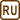## Charge Transferred Through a Conductor

### Task number: 1790

What electric charge is transferred through a conductor over $$10\,\mathrm{s}$$, when:

1. a constant current of $$5\,\mathrm{A}$$ flows through the contuctor
2. the current through the conductor increases steadily from $$0\,\mathrm{A}$$ to $$3\,\mathrm{A}$$.
• #### Notation

 $$t = 10\,\mathrm{s}$$ Time during which the current flows through the conductor $$I = 5\,\mathrm{A}$$ Constant current $$I_1 = 0\,\mathrm{A}$$ Starting current $$I_2 = 3\,\mathrm{A}$$ Final current $$Q = ?\,\mathrm{[C]}$$ Transferred charge
• #### Hint I.

What is the relation between current, charge and time?

• #### Solution I.

For the constant current, we calculate the charge from the formula

$I=\frac{Q}{t}.$

The charge Q transferred by the current I over time t is then

$Q = I \cdot t = 5\,\mathrm{s} \,\cdot\, 10\,\mathrm{A}= 50\,\mathrm{C}.$
• #### Hint II.

The current is not constant in the second case. Try to draw the graph of current versus time and think if you can assess the transferred charge from this graph.

• #### Solution II.

The total charge transferred by the steadily increasing current is:

$Q=I_\mathrm{ave} \cdot t = \frac{I_1 + I_2}{2}t=\frac{0+3}{2}\cdot10= 15\,\mathrm C,$

where Iave is the average of the inital current I1 and the final current I2.

• #### Answer

1. A charge of $$50\, \mathrm C$$ is transferred through a conductor by the current $$5\,\mathrm A$$ over $$10\,\mathrm s$$.
2. A charge of $$15\,\mathrm C$$ is transferred with the current that steadily increases from zero value to $$3\,\mathrm A$$over the same time.